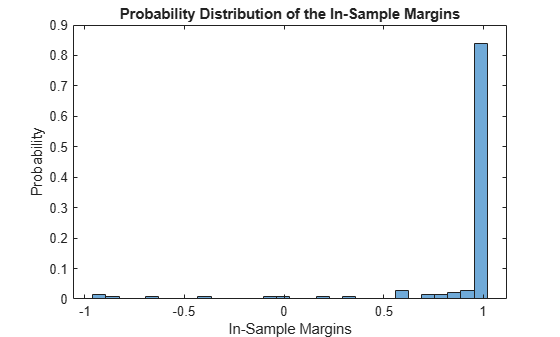Documentation

# resubMargin

Class: ClassificationNaiveBayes

Classification margins for naive Bayes classifiers by resubstitution

## Syntax

``m = resubMargin(Mdl)``

## Description

example

````m = resubMargin(Mdl)` returns the resubstitution classification margins (`m`) for the naive Bayes classifier `Mdl` using the training data stored in `Mdl.X` and corresponding class labels stored in `Mdl.Y`.```

## Input Arguments

expand all

A fully trained naive Bayes classifier, specified as a `ClassificationNaiveBayes` model trained by `fitcnb`.

## Output Arguments

expand all

Classification margins, returned as a numeric vector.

`m` has the same length equal to `size(Mdl.X,1)`. Each entry of `m` is the classification margin of the corresponding observation (row) of `Mdl.X` and element of `Mdl.Y`.

## Examples

expand all

```load fisheriris X = meas; % Predictors Y = species; % Response```

Train a naive Bayes classifier. It is good practice to specify the class order. Assume that each predictor is conditionally, normally distributed given its label.

`Mdl = fitcnb(X,Y,'ClassNames',{'setosa','versicolor','virginica'});`

`Mdl` is a `ClassificationNaiveBayes` classifier.

Estimate the in-sample classification margins. Display the distribution of the margins using a boxplot.

```m = resubMargin(Mdl); figure; boxplot(m); h = gca; iqr = quantile(m,0.75) - quantile(m,0.25); h.YLim = median(m) + iqr*[-4 4]; title 'Boxplot of the Margins';```An observation margin is the observed (true) class score minus the maximum false class score among all scores in the respective class. Classifiers that yield relatively large margins are desirable.

The classifier margins measure, for each observation, the difference between the true class observed score and the maximal false class score for a particular class. One way to perform feature selection is to compare in-sample margins from multiple models. Based solely on this criterion, the model with the highest margins is the best model.

Load Fisher's iris data set. Define two data sets:

• `fullX` contains all predictors (except the removed column of 0s).

• `partX` contains the last 20 predictors.

```load fisheriris X = meas; % Predictors Y = species; % Response fullX = X; partX = X(:,3:4);```

Train naive Bayes classifiers for each predictor set.

```FullMdl = fitcnb(fullX,Y); PartMdl = fitcnb(partX,Y);```

Estimate the in-sample margins for each classifier. Compute confidence intervals for each sample.

```fullM = resubMargin(FullMdl); partM = resubMargin(PartMdl); n = size(X,1); fullMCI = mean(fullM) + 2*[-std(fullM)/n std(fullM)/n]```
```fullMCI = 1×2 0.8898 0.8991 ```
`partMCI = mean(partM) + 2*[-std(partM)/n std(partM)/n]`
```partMCI = 1×2 0.9129 0.9209 ```

The confidence intervals are tight, and mutually exclusive. The margin confidence interval of the classifier trained using just predictors 3 and 4 has higher values than that of the full model. Therefore, the model trained on two predictors has better in-sample performance.